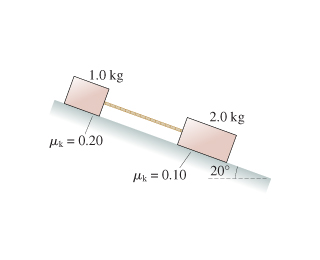# Problem: The two blocks in the figure (Figure 1) are sliding down the incline. What is the tension in the massless string?

###### FREE Expert Solution

For force problems, we always use Newton's second law:

$\overline{){\mathbf{\Sigma }}{\mathbf{F}}{\mathbf{=}}{\mathbf{m}}{\mathbf{a}}}$

90% (279 ratings)###### Problem DetailsThe two blocks in the figure (Figure 1) are sliding down the incline. What is the tension in the massless string?